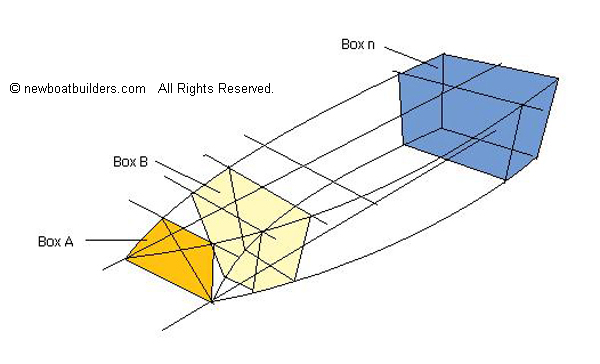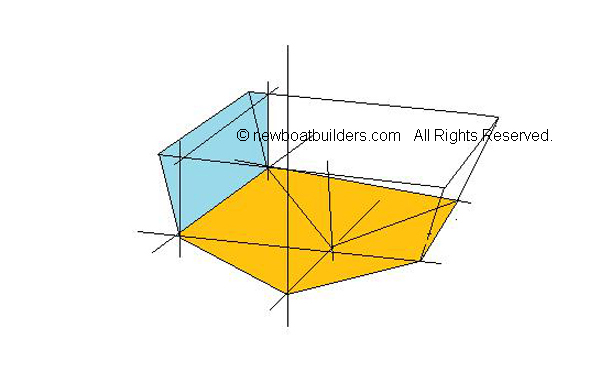MENU Resize to Fit-Double Tap ScreenEverything Boat Building
Don't tell me that I can't. Tell me how I can.
Disclaimer:   I am not a spokesperson for the US Coast Guard or ABYC. For an official interpretation of regulations or standards you must contact the US Coast Guard or other organization referenced..   More.....
 I developed an E-course in Capacity and Flotation for Boat Builders, for Professional Boat Builder Magazine. They no longer offer it.  In addition to these web pages  I am offering it as an e-book. Go to Ike's Store to see more I also have a perfect companion to the Capacity and Flotation Course.  It is an excel spreadsheet that does the calculations to determine the amount of Flotation needed. Easy to use. Go to Ike's Store to see more

## Methods Of Calculating Flotation

### Flotation regulations for recreational boats.  How to calculate flotation amounts.

One of the more important tasks a builder must perform for a small boat is determining weight and persons capacity, calculating how much flotation is needed and making a label for the boat. Also attaching a Hull Identification Number (HIN) is required.

Back Yard Boat Builders (Home Built): HINs

For back yard boat builders in the US, who are building a boat for themselves, an HIN can be obtained from the state office that registers boats.  When registering the boat tell them it is a home built boat. They will have you fill out a form declaring that you built the boat, and assign an HIN.

In Canada this number is available from the Canadian Office of Boating Safety:

Calculations:

Methods of Calculating Capacity:

Many people consider calculating capacities and flotation as some mysterious scientific process beyond the capabilities of the average boat builder. This is definitely not true. Anyone with a basic education can do it.  If you can add and subtract, multiply and divide, you can calculate boat capacities and flotation.  For a boat like the FL 12 it is even simpler because the shapes are all squares, rectangles and triangles. You need to be able to find area and volume.

Area of any rectangle is the length times width.

Area of a square is one side times itself (or length times width but in this case the length equals the width)

The area of a triangle equals the base of the triangle (the bottom width) times the height, divided by two.

Volume involves one more dimension, the thickness or depth. So the volume of a box is the length of the box times the width times the depth.

The same applies to a triangular shapes.

Any boat like the FL12 that has a flat bottom, or even a vee bottom can be divided up into these shapes and the volume of each shape is found.  Then the volumes are added up.  The total volume times the weight of water (62.4 pounds per cubic foot) equals the displacement.

As shown in the below illustration the volume of this shape is relatively simple to calculate by simplifying the shape and taking each piece at a time.Length W1 + W2 divided by 2 is the average width, W, of the front side of the box.

(W1 + W2)/2 times height b gives the area of the front of the box,

Find the area of the back face using the same method.

Then add the two areas together and divide by two to get the average area.  Multiply the average area by the width of the box (length e) to get the volume of the box.

By doing this for each section of the boat, and then adding up the volumes, you get the total volume of the boat.

Displacement is the weight of the water that is moved out of the way when you make a hole in the water by putting a boat in it. Displacement divided by five is the maximum weight capacity.  If you subtract the weight of the outboard motor, battery, and a portable fuel tank, you get maximum persons capacity.  That weight plus 32 and divided by 141 equals the number of person.

Of course the simplest way to do this is put weight in the boat.  Add weights until water is about to come in. The total weight is the displacement weight. Divide the total by five, and that gives you maximum weight capacity. Subtract the weight of the outboard motor, battery, and a portable fuel tank and you have persons weight.  Add 32 to persons weight and divide by 141 to get persons.

An easy way to find persons capacity is to put weight on one side of the boat as far outboard as possible.  Add weight until the boat heels to the point where water comes in.  Divide that weight by 0.6 (or multiply by 1.6667) and that is the maximum persons Weight.

Add the engine weight, battery, and a portable fuel tank, to the Maximum Persons Weight and multiply by five and you have the Maximum Weight Capacity.

For the FL 12 I divided the boat into 10 equal sections. Each section forms a box.  Then I measured the dimensions of each box to get the volume.

Starting at the bow. The bow section, shown here as Box A, forms a triangular shaped box. Box A is 10” by 18 ½” by 21 ½”10” = 0.8333 ft

18 ½ “ = 1.54 ft

21 ½  = 1.79 ft

The formula is 0.8333 X 1.54 / 2 = 0.642

Then multiply 1.79 X 0.642 / 2 = 0.5746  = volume of Box 1

Box B is a little more complicated because one side is large than the other side.  That is the rear side is bigger than the front side.

The front side is 21 ½ by 18 ¼ by 11 ½.  It is not a rectangle, but since two sides are the same length we can treat it the same as a rectangle by using the average of the length of the two unequal sides.

21 + 11 ½ = 32 ½   32 ½  / 2 = 16 ¼ = 1.354 front area

the rear side = 28 ¾ by 22 by 18 ½

28 ¾  + 22 = 50 ¾   50 ¾ / 2 = 25 3/8 = 2.115 rear area

 Station Volume bow 0.2535 0-1 1.3940 1-2 2.6700 2-3 3.5800 3-4 4.3570 4-5 4.7700 5-6 4.8370 6-7 4.7990 7-8 4.5380 8-9 4.1670 9-10 3.6400 10-T 1.7483 Total Vol 40.7538 disp lb 2543.0371

Then divide the displacement weight by five to get the maximum weight capacity.  Subtract the weight of the outboard motor for persons weight.  Get the weight of the motor from USCG Table 4 or ABYC S-10. To get the number of people add 32 to the persons weight and divide by 141.

But I wanted to see how this compares to doing it by using Simpson's Formula to see how accurate it is.

By using both methods the results were very close to each other, and the number of persons was the same.

 Weights are in pounds Simpsons Blocks Boat Weight 100 100 Max Disp- Boat Weight 2445.30 2443.04 Max Weight Capacity 733.59 732.91 Max Persons Capacity 637.73 637.12 Max Persons 4.75 4.75

This method is not limited to flat bottom boats.  Boats with more complex shapes can use the same method.  The following illustrates a vee bottom boat.Each block can be split into triangles, rectangles and squares.  The areas and volumes are simple to calculate.  Curves to the shape can be considered as straight lines if you break it into smaller blocks.

Flotation Calculations:

Once you know the capacities calculating flotation is next.

You need to find the amount of flotation to float three things;

The boat itself:

The persons:

The engine (if there is one.):

Wood Boats:

Example:  The boat is plywood.  The boat weighs 100 lbs. Plywood is buoyant so the amount needed is negative, that is the plywood provides some flotation. Flotation foam at 2 lbs per cubic foot supports 60.4 lbs.

Boat Weight (Wh) X a factor for plywood (-0.81) divided by 60.4 = Cubic feet = -1.34

The outboard motor:  a 2HP motor weighs 30 lbs according to

Submerged it weighs 27 lb.  For a 2 HP motor there is no battery or fuel tank weight.

Engine Weight (27) divided by 60.4 = 0.44701 (0.45) Cu Ft

The people. People don't weigh as much in water as they do in air. Plus that it is assumed that with flotation, part of the persons body will be out of the water so the factor is 2/15.  But in the USCG Guidelines they use 1/4, or 0.25.  This gives you a safety factor of about two for the amount of flotation for persons weight.

Persons weight (627.72 x 0.25) divided by 60.4 equals 2.639611

Total Flotation = 0.45 + 2.639611 - 1.34106 = 1.74855 (1.8) Cu Ft

Round this to 1.75, 1.8  or even two.

If the boat is made of fiberglass the the calculation for flotation for the boat has to include weight of the Fiberglass, which is not buoyant like wood.

Boat Weight (Wh) X a factor for Fiberglass (0.33), divided by 60.4 = Cubic feet  of Flotation

If the boat weighs 100 lb and is all fiberglass;

Flotation for the boat = (100 x 0.33)/60.4 = 0.546 (0.6) Cubic Ft.

Manually propelled Boats:

However, if this is a manually propelled boat, i.e. no motor, Engine weight is zero. engine weight is taken out of the equation.

Boat Weight (Wh) X a factor for plywood (-0.81) divided by 60.4 = Cubic feet = -1.34

Persons weight (627.72 x 0.25) divided by 60.4 equals 2.639611

Total Flotation = 2.639611 - 1.34106 = 1.29855 (1.3) Cu Ft

Air Chambers for Flotation:

Suppose the boat uses air chambers for flotation? Then you would divide by 62.4 rather than 60.4  because in these calculations air has no weight and supports 62.4 lb per cubic foot in fresh water.

Where to put the Flotation.

Place the flotation for the engine in the back of the boat on the inner side of the transom. Split it in two and put half in the starboard quarter and the other in the port quarter. This supports and balances the engine weight.

Divide the persons flotation up and put it under the seats so that it will stabilize the boat when it is swamped.